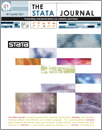Home  >>  Archives  >>  Volume 6 Number 4  >>  st0116

#### The Stata JournalVolume 6 Number 4: pp. 561-579

Subscribe to the Stata Journal## Speaking Stata: In praise of trigonometric predictors

 Nicholas J. Cox Department of Geography Durham University Durham City, UK n.j.cox@durham.ac.uk
Abstract.   Using sine and cosine terms as predictors in modeling periodic time series and other kinds of periodic responses is a long-established technique, but it is often overlooked in many courses or textbooks. Such trigonometric regression is straightforward in Stata through applications of existing commands. I give various examples using classic periodic datasets on the motion of the asteroid Pallas and the daily rhythm of birth numbers. I make a brief connection to polynomial-trigonometric regression.

View all articles by this author: Nicholas J. Cox

View all articles with these keywords: circular regression, Fourier regression, harmonic regression, periodic regression, polynomial-trigonometric regression, trigonometric regression, sine, cosine